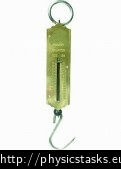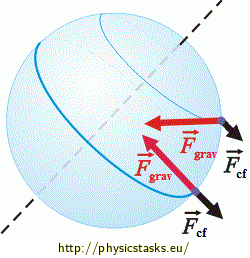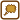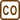## Spring Scale on the Pole and on the Equator

We calibrated a spring scale on the North Pole and then we moved the scale to the Equator.

Does the spring scale give the same readings as on the pole? Give reasons.

• #### Hint 1 - Principle of operation of the spring scale

What is the principle of the operation of the spring scale? What physical quantity does it actually measure?• #### Hint 2 - What is the weight comprised of?

The weight of an object arises from (and its magnitude is affected by) two other forces. What are these two forces? In what directions do they act?

• #### Hint 3 - Gravity and centrifugal force

We already know that the magnitude of the weight (often denoted simply as scalarW) depends on the magnitude of the true gravity and on the magnitude of the centrifugal force.

Is the magnitude of the true gravity (acting on a given object) the same everywhere on the surface of the Earth? If not so, where is it the greatest and where is it the smallest?

Is the magnitude of the centrifugal force (acting on a given object) the same everywhere on the surface of the Earth? If not so, where is it the greatest one and where is it the smallest one?

• #### Hint 4

What follows from the previous hints regarding the magnitude of the weight on the poles and on the Equator (and also regarding the readings measured by the spring scale there)? Where does the spring scale indicate a larger weigth?
• #### Complete solution

The spring scale measures the weight $$\vec{W}$$ - the force that the object acts on the spring in the reference frame of the scale.

The scale and the object are both located in the non-inertial reference frame of the Earth that is rotating around its axis with the angular velocity $$\omega$$. The weight is composed of:

1) the true gravity $$\vec{F}_{grav}$$ acting between the Earth and the object - this force points towards the Earth center and its magnitude is the same everywhere on the surface of the Earth, according to Newton’s law of universal gravitation:

$F_{grav}=G\frac{m\,M_E}{R_E^2}\,,$

where G is the gravitational constant, m is the mass of the object, $$M_E$$ is the Earth mass and $$R_E$$ is the distance between the object and the Earth’s center - the Earth radius (we suppose that the Earth is a sphere).

2) the centrifugal force $$\vec{F}_{cf}$$ that arises due to the rotation of the Earth around its axis (it is an inertial or fictitious force, one cannot find who or what body is its originator). Its vector lies in the plane perpendicular to the axis of the rotation (the Earth axis) and its magnitude depends on the position on the surface of the Earth according to the formula:

$F_{cf}=m{\omega^2}r=m\left(\frac{2\pi}{T}\right)^{2}r,$

where m is the mass of the object, $$\omega$$ is the angular velocity of rotation, T is the period of one turn of the Earth around its axis and r is the radius of the circle on which the given point revolves with respect to the axis of rotation (it is the full Earth radius at the Equator only).Together: $\vec{W}=\vec{F}_{grav}+\vec{F}_{cf}$

On the Pole, there is r = 0 and the centrifugal force is zero. The weight is then identical to the true gravitational force as for both the direction and the magnitude:

$\vec{W}_{Pole}=\vec{F}_{grav}$ $W_{Pole}=F_{grav}$

On the Equator, there is a maximum centrifugal force that aims in the opposite direction than the true gravity (see the Figure):

$\vec{W}_{Eq}=\vec{F}_{grav}+\vec{F}_{cf}$ $W_{Eq}=F_{grav}-F_{cf}$

Since the spring scale measures the weight, it indicates a smaller mass on the Equator than on the (North) Pole.

For an object with a mass of 1 kg:

$M_E=5.98{\cdot}10^{24}\,\mathrm{kg}$ $R_E=6.378{\cdot}10^{6}\,\mathrm{m}$ $G=6.67{\cdot}10^{-11}\,\mathrm{N\cdot{m^2}\cdot{kg^{-2}}}$ $T=24\,\mathrm{h}=86400\,\mathrm{s}$

$W_{Pole}=G\frac{m\,M_E}{R_E^2} = \left\{ 6.67{\cdot}10^{-11}\cdot\frac{1{\cdot}5.98{\cdot}10^{24}}{(6.378{\cdot}10^6)^2}\right\}\,\mathrm{N}\,\dot{=}\,9.805\,\mathrm{N}$

\begin{align}W_{Eq} &=G\frac{m\,M_E}{R_E^2}-m\left(\frac{2\pi}{T}\right)^{2}R_E=\\ &=\left\{6.67{\cdot}10^{-11}\cdot\frac{1{\cdot}5.98{\cdot}10^{24}}{(6.378{\cdot}10^6)^2}-1\cdot\left(\frac{2\pi}{86400}\right)^2\cdot{6.378{\cdot}10^6}\right\}\,\mathrm{N}\,\dot{=}\\\\&\,\dot{=}\,\left(9.8052-0.0337\right)\,\mathrm{N} \,\dot{=}\, 9.772\,\mathrm{N}\end{align}

The spring scale indicates a smaller weight on the Equator than on the Pole when weighing the same object.

• #### The actual reading on the Equator

A spring scale that was calibrated on the Pole indicates a mass of 1 kg when its spring is elongated by a force of 9.805 N - the weight of a 1–kg–object on the Pole.

The same object elongates the spring of the scale by a force of 9.771 N on the Equator and the Pole–calibrated scale thus indicates a mass of 9.772/9.805 = 0.997 kg here.